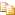﻿AddRange Method (array, arrayIndex, count)AvalonEdit
Appends new elements to the end of this rope. Runs in O(lg N + M), where N is the length of this rope and M is the number of new elements.Declaration Syntax
 C# Visual Basic Visual C++
```public void AddRange(
T[] array,
int arrayIndex,
int count
)```
```Public Sub AddRange ( _
array As T(), _
arrayIndex As Integer, _
count As Integer _
)```
```public:ParametersExceptions